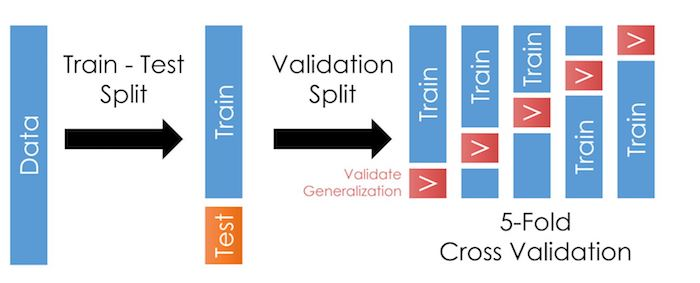# 5.4.3. Cross-Validation¶

Cross-validation is a step where we take our training sample and further divide it in many folds, as in the illustration here:As we talked about in the last chapter, cross-validation allows us to test our models outside the training data more often. This trick reduces the likelihood of overfitting and improves generalization: It should improve our model’s performance when we apply it outside the training data.

Warning

I say “should” because the exact manner in which you create the folds matters.

• If your data has groups (i.e. repeated observations for a given firm), you should use group-wise cross-validation, like `GroupKFold` to make sure no group is in the training and validation partitions of the fold

• If your data and/or task is time dependent, like predicting stock returns, you should use a time-wise cross-validation, like `TimeSeriesSplit` to ensure that the validation partitions are subsequent to the training sample

## 5.4.3.1. CV in practice¶

Like before, let’s load the data. Notice I consolidated the import lines at the top.

```import pandas as pd
import numpy as np
from sklearn.linear_model import Ridge
from sklearn.model_selection import train_test_split

url        = 'https://github.com/LeDataSciFi/ledatascifi-2021/blob/main/data/Fannie_Mae_Plus_Data.gzip?raw=true'
y          = fannie_mae.Original_Interest_Rate
fannie_mae = (fannie_mae
.assign(l_credscore = np.log(fannie_mae['Borrower_Credit_Score_at_Origination']),
l_LTV = np.log(fannie_mae['Original_LTV_(OLTV)']),
)
.iloc[:,-11:] # limit to these vars for the sake of this example
)
```

### 5.4.3.1.1. STEP 1: Set up your test and train split samples¶

```rng = np.random.RandomState(0) # this helps us control the randomness so we can reproduce results exactly
X_train, X_test, y_train, y_test = train_test_split(fannie_mae, y, random_state=rng)
```

An important digression: Now that we’ve introduced some of the conceptual issues with how you create folds for CV, let’s revisit this `test_train_split` code above. This page says `train_test_split` uses ShuffleSplit. This method does not divide by time or any group type.

If you want to use any other CV iterators to divide up your sample, you can:

```# Just replace "GroupShuffleSplit" with your CV of choice,
# and update the contents of split() as needed

train_idx, test_idx = next(
GroupShuffleSplit(random_state=7).split(X, y, groups)
)
X_train, X_test, y_train, y_test = X[train_idx], X[train_idx], y[test_idx], y[test_idx]
```

Back to our regularly scheduled “CV in Practice” programming.

### 5.4.3.1.2. STEP 2: Set up the CV¶

SK-learn makes cross-validation pretty easy. The `cross_validate("estimator",X_train,y_train,cv,scoring,...)` function (documentation here) will

1. Create folds in X_train and y_train using the method you put in the `cv` parameter. For each fold, it will create a smaller “training partition” and “testing partition” like in the figure at the top of this page.

2. For each fold,

1. It will fit your “estimator” (as if you ran `estimator.fit(X_trainingpartition,y_trainingpartition)`) on the smaller training partition it creates. Your estimator will be a “pipeline” object (covered in detail on the next page) that tells sklearn to apply a series of steps to the data (preprocessing, etc.), culminating in a model.

2. Use that fitted estimator on the testing partition (as if you ran `estimator.predict(X_testingpartition)` will apply all of the data transformations in the pipeline and use the estimated model on it)

3. Score those predictions with the function(s) you put in `scoring`

3. Output a dictionary object with performance data

You can even give it multiple scoring metrics to evaluate.

So, you need to set up

1. Your preferred folding method (and number of folds)

3. Your scoring method (you can specify this inside the cross_validate function)

```from sklearn.model_selection import KFold, cross_validate

cv = KFold(5)                          # set up fold method
ridge = Ridge(alpha=1.0)               # set up model/estimator
cross_validate(ridge,X_train,y_train,
cv=cv, scoring='r2')     # tell it the scoring method here
```
```{'fit_time': array([0.01695871, 0.00604463, 0.00346589, 0.0063622 , 0.00415468]),
'score_time': array([0.00298142, 0.0017314 , 0.00103474, 0.0025425 , 0.        ]),
'test_score': array([0.90789446, 0.89926394, 0.900032  , 0.90479828, 0.90327986])}
```

Note

Wow, that was easy! Just 3 lines of code (and an import)

And we can output test score statistics like:

```scores = cross_validate(ridge,X_train,y_train,cv=cv, scoring='r2')
print(scores['test_score'].mean()) # scores is just a dictionary
print(scores['test_score'].std())
```
```0.9030537085469961
0.003162930786979486
```

## 5.4.3.2. Next step: Pipelines¶

The model above

• Only uses a few continuous variables: what if we want to include other variable types (like categorical)?

• Uses the variables as given: ML algorithms often need you to transform your variables

• Doesn’t deal with any data problems (e.g. missing values or outliers)

• Doesn’t create any interaction terms or polynomial transformations

• Uses every variable I give it: But if your input data had 400 variables, you’d be in danger of overfitting!

At this point, you are capable of solving all of these problems. (For example, you could clean the data in pandas.)

But for our models to be robust to evil monsters like “data leakage”, we need the fixes to be done within pipelines.